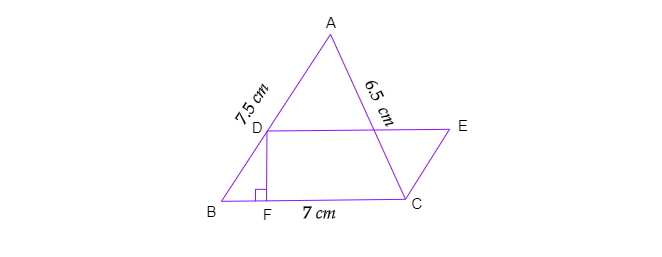"
">

# In the given figure, $\triangle ABC$ has sides $AB=7.5\ cm$, $AC=6.5\ cm$ and $BC=7\ cm$. On base $BC$, a parallelogram $DBCE$ of same area that of $\vartriangle ABC$ is constructed. Find the height $DF$ of the parallelogram."

Given: In the given figure, $\triangle ABC$ has sides $AB=7.5\ cm$, $AC=6.5\ cm$ and $BC=7\ cm$. On base $BC$, a parallelogram $DBCE$ of same area that of $\vartriangle ABC$ is constructed.

To do: To find the height $DF$ of the parallelogram.

Solution:

Here, $a=7.5\ cm,\ b=6.5\ cm$ and $c=7\ cm$

$s=\frac{a+b+c}{2}$

$=\frac{7.5+6.5+7}{2}$

$=10.5$

Area of $\vartriangle ABC$,

$A=\sqrt{s( s-a)( s-b)( s-c)}=\sqrt{10.5( 10.5−7.5)( 10.5−6.5)( 10.5−7)}$

$=\sqrt{441}$

$=21\ cm^2$

Area of parallelogram $BDEC=$ Area of $\vartriangle ABC$

$\Rightarrow$ Base $\times$ Height$=21$

$\Rightarrow 7\times DF=21$

$\Rightarrow DF=\frac{21}{7}$

$\Rightarrow DF=3\ cm$

Thus, height of the parallelogram $DBCE$ is $DF=3\ cm$.

Updated on: 10-Oct-2022

17 Views